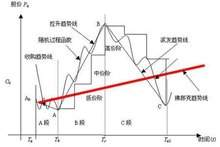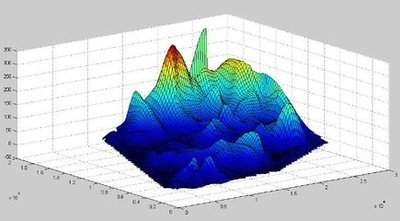Language :
 Search
 Picture : Type :[|jpg|gif|jpeg|png|] Byte :[＜2000KB] Language : 中文(简体) 中文(繁體) English 日本 한국어 Deutsch Française Ελληνικά Россию svenska Nederlandse Polska Український dansk български Italiano Icelandic român suomen kieli Galicia Türk Pilipino Català český hrvatski Latvijas Lietuvos македонски norsk språk Српски језик slovenský jazyk slovenščina Magyar nyelv فارسی Português ไทย Español Bahasa Indonesia Ngôn ngữ Việt Nam العربية Gaeilge shqiptar eesti Беларускія Die Boole-taal (Afrikaans) Malti Melayu lugha ya Kiswahili Cymraeg עברית שפה ייִדיש हिन्दी esperanto bosanski اردو زبان Azərbaycan ქართული Kreyòl ayisyen Euskal հայերեն ગુજરાતી ಕನ್ನಡ latin ພາສາລາວ বাংলা ভাষা తెలుగు தமிழ் மொழி ខ្មែរ | Check code :[Visitor (112.21.*.*)]answers [Chinese ] Time :2022-03-20At present, mathematical models do not yet have a uniform and accurate definition, because different perspectives can have different definitions. However, we can give the following definition. A mathematical model is an abstract, simplified structure about parts of the real world and for a particular purpose. Specifically, mathematical models are equations or inequalities established with letters, numbers, and other mathematical symbols for some purpose, as well as mathematical structural expressions in which charts, images, block diagrams, etc. describe the characteristics of objective things and their intrinsic connections.Mathematical modeling: It is a new discipline developed in recent years, and it is a science that combines mathematical theory with practical problems. It reduces real-world problems to corresponding mathematical problems, and on this basis uses mathematical concepts, methods and theories for in-depth analysis and research, so as to portray practical problems from a qualitative or quantitative point of view, and provide accurate data or reliable guidance for solving real-world problems.
 [Visitor (112.21.*.*)]answers [Chinese ] Time :2022-03-20Mathematical modelingIn the past half century, with the rapid development of computer technology, the application of mathematics has not only played an increasingly important role in engineering technology, natural sciences and other fields, but also penetrated into new fields such as economy, management, finance, biology, medicine, environment, geology, population, and transportation with unprecedented breadth and depth, and the so-called mathematical technology has become an important part of contemporary high and new technologies.A mathematical model is a simulation, which is an abstract and concise portrayal of the essential properties of the actual subject with mathematical symbols, mathematical formulas, programs, graphs, etc., which can either explain some objective phenomena, or predict future development laws, or provide an optimal strategy or a better strategy in a certain sense for controlling the development of a certain phenomenon. Mathematical models are generally not direct copies of real-world problems, and their establishment often requires both in-depth and subtle observation and analysis of real-world problems and the flexible and skillful use of various mathematical knowledge. This process of applying knowledge to abstract and extract mathematical models from practical topics is called mathematical modeling.Whether it is a mathematical method to solve a practical problem in the field of science and technology and production, or a combination with other disciplines to form an interdisciplinary discipline, the first and key step is to establish a mathematical model of the object of study and calculate it to solve (usually with the help of computers); The role of mathematical modeling and computer technology in the era of the knowledge economy can be described as a tiger.